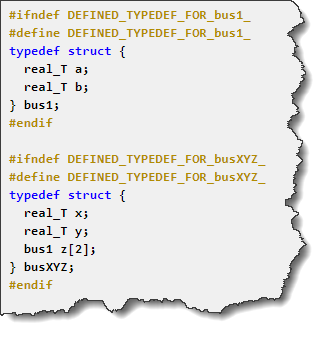# Array of Buses and S-Function Builder support

This week, Navid Rahbariasr proposed to publish a blog post about support for arrays of buses being added to the S-Function Builder in MATLAB R2021b.
This made me realize that I have never covered the fundamental of array of buses in Simulink. So, this week we will walk you through the basics of creating arrays of buses, and conclude by describing how they can now be used in the S-Function Builder block in MATLAB R2021b.

## The MATLAB Equivalent: Structure Arrays

In MATLAB, it is possible to create a structure array using code like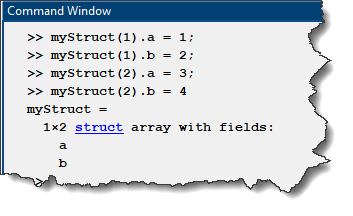Another equivalent code could be: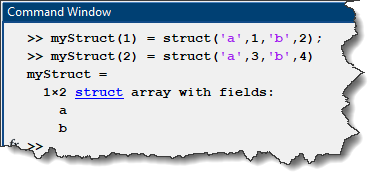Let's see how we can do the same in Simulink.

## Creating an Array of Buses

In Simulink, the equivalent of a MATLAB structure is a bus signal. So, let's try to create two identical bus signals and concatenate them into an array using a Vector Concatenate block, here is what the model looks like after updating the diagram:If you are familiar with signal line styles in Simulink, you probably already concluded that there is something not working as expected here. The two signals coming out of Bus Creator 1 and Bus Creator 2 are buses, but the output of the Vector Concatenate is a vector of 4 elements, not an array of buses.
The reason is that Simulink can treat bus signals as vector. In this case, Simulink decides to treat the bus signals as vector is because they are virtual buses. Only nonvirtual buses can be concatenated into an array of buses.
To make the bus signals nonvirtual, it is possible to use Simulink.Bus.createObject or the Bus Editor to create a bus object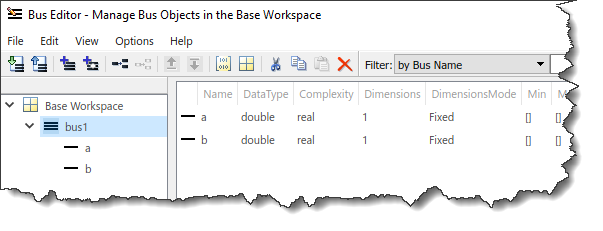This bus object can then be used in Bus Creator blocks to specify that the output should be nonvirtual: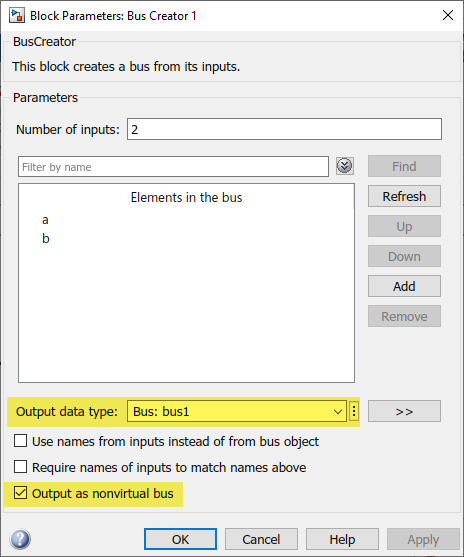and now the signal lines styles are as expected, we are producing an Array of two buses.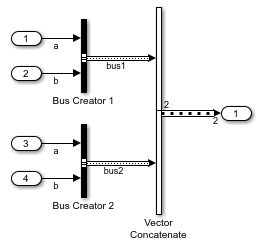Note: If you would like to avoid Simulink treating bus signal as vector and instead prefer getting a warning or an error, look at the diagnostic Bus signal treated as vector

## Nested Array of Buses

Let's extend our example by including the array of buses previously created into a larger bus: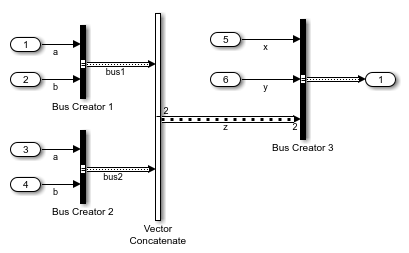To make that happen, element "z" of the new bus object must be of type "bus1" and of dimension 2.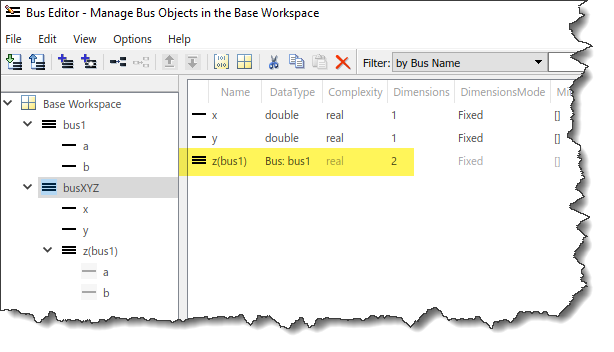## Accessing Arrays of Buses in MATLAB Function block and Stateflow

When feeding a bus or array of buses to a MATLAB Function block or a Stateflow chart, it is possible to access the individual elements just like a structure array in MATLAB: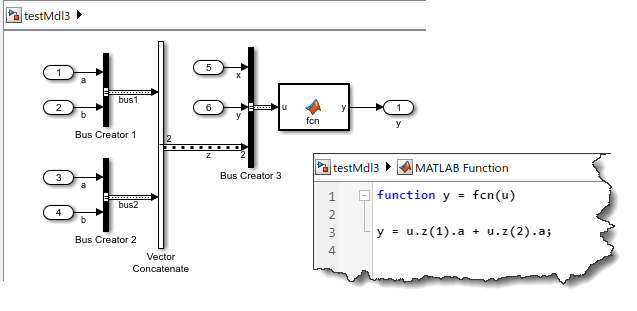By the way, in case you had not noticed, in MATLAB R2021b, the code for MATLAB Function blocks now opens embedded in the Simulink canvas instead of the MATLAB Editor.

## Accessing Arrays of Buses in S-Function Builder

As mentioned at the beginning of this post, in MATLAB R2021b support for nested arrays of buses has been added to the S-Function Builder block.
Let's replace the MATLAB Function block by the S-Function Builder in the previous model:For the block to accept the bus signal, we first need to specify the corresponding bus object in the Ports and Parameters panel. Then you can type the C code equivalent to the MATLAB code above in the Outputs section of the S-function Builder app. Note that u0 is a pointer type, so we need to dereference it using "->" to get to the elements. Also C-Language uses zero based indexing, so the first element of z would be z: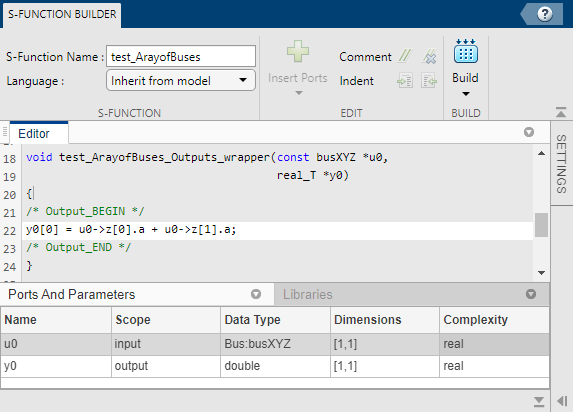After building the S-Function, you should notice a header file with the bus definitions in the current directory: